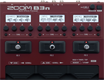# myMMStingrayClassic

Discussion in 'Zoom B3n' started by Thomas, May 22, 2019.

1. myMMStingrayClassicDevice: Zoom B3n
Firmware: 2.00

Name on device: Musicman
Optimized for: Phones/Speaker

Effects chain:Style of Musicman

Effect: "BlackOpt" (Dynamics), active - "yes"
"Comp" = 65
"Lo" = 59
"Hi" = 63
"Volume" = 76

Effect: "BassGEQ" (Filter), active - "yes"
"50Hz" = 2.0
"120Hz" = 3.0
"400Hz" = 2.0
"500Hz" = 0.0
"800Hz" = 2.0
"4.5kHz" = 0.0
"10kHz" = 2.0
"Volume" = 80
"50Hz" = 0.0
"120Hz" = 0.0
"400Hz" = 0.0
"500Hz" = 0.0
"800Hz" = 0.0
"4.5kHz" = 0.0
"10kHz" = 0.0
"Volume" = 80
"Parameter set" = A

Effect: "AG 750" (Amp simulator), active - "yes"
"Bass" = 41
"Middle" = 56
"Treble" = 50
"Gain" = 50
"Bright" = On
"Deep" = Off
"Volume" = 58
"SOLO" = 1

Effect: "SMR4x10TW" (Cabinet), active - "yes"
"DYN20" = 21
"DYN57" = 43
"Bottom" = 55
"Balance" = 28

Patch Volume: 100